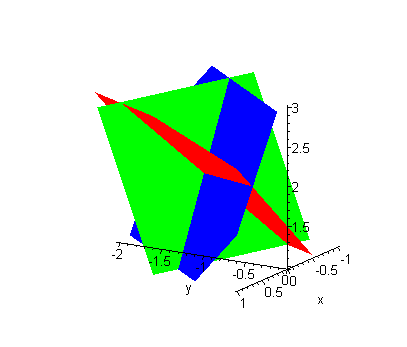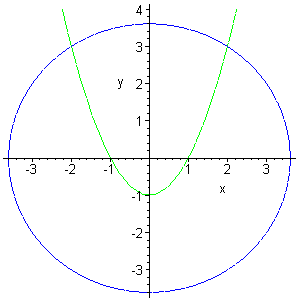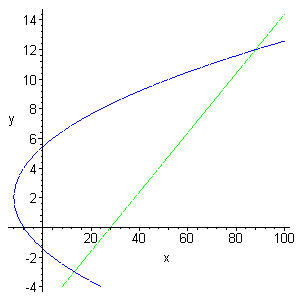Paul's Online Notes
Home / Algebra Trig Review / Algebra / Solving Systems of Equations
Show Mobile Notice Show All Notes Hide All Notes
Mobile Notice
You appear to be on a device with a "narrow" screen width (i.e. you are probably on a mobile phone). Due to the nature of the mathematics on this site it is best views in landscape mode. If your device is not in landscape mode many of the equations will run off the side of your device (should be able to scroll to see them) and some of the menu items will be cut off due to the narrow screen width.

### Solving Systems of Equations

Show All Solutions Hide All Solutions

1. Solve the following system of equations. Interpret the solution.

\begin{align*}2x - y - 2z & = - 3\\ x + 3y + z & = - 1\\ 5x - 4y + 3z & = 10\end{align*} Show Solution

There are many possible ways to proceed in the solution process to this problem. All will give the same solution and all involve eliminating one of the variables and getting down to a system of two equations in two unknowns which you can then solve.

#### Method 1

The first solution method involves solving one of the three original equations for one of the variables. Substitute this into the other two equations. This will yield two equations in two unknowns that can be solve fairly quickly.

For this problem we’ll solve the second equation for $$x$$ to get.

$x = - z - 3y - 1$

Plugging this into the first and third equation gives the following system of two equations.

\begin{align*}2\left( { - z - 3y - 1} \right) - y - 2z & = - 3\\ 5\left( { - z - 3y - 1} \right) - 4y + 3z & = 10\end{align*}

Or, upon simplification

\begin{align*} - 7y - 4z & = - 1\\ - 19y - 2z &= 15\end{align*}

Multiply the second equation by -2 and add.

\begin{align*} - 7y - 4z & = - 1\\ 38y + 4z & = - 30\\ \hline 31y & = - 31\end{align*}

From this we see that $$y = - 1$$. Plugging this into either of the above two equations yields $$z = 2$$. Finally, plugging both of these answers into $x = - z - 3y - 1$ yields $$x = 0$$.

#### Method 2

In the second method we add multiples of two equations together in such a way to eliminate one of the variables. We’ll do it using two different sets of equations eliminating the same variable in both. This will give a system of two equations in two unknowns which we can solve.

So, we’ll start by noticing that if we multiply the second equation by -2 and add it to the first equation we get.

\begin{align*}2x - y - 2z & = - 3\\ - 2x - 6y - 2z & = 2\\ \hline - 7y - 4z & = - 1\end{align*}

Next multiply the second equation by -5 and add it to the third equation. This gives

\begin{align*} - 5x - 15y - 5z & = 5\\ 5x - 4y + 3z & = 10\\ \hline - 19y - 2z & = 15\end{align*}

This gives the following system of two equations.

\begin{align*} - 7y - 4z & = - 1\\ - 19y - 2z & = 15\end{align*}

We can now solve this by multiplying the second by -2 and adding

\begin{align*} - 7y - 4z & = - 1\\ 38y + 4z & = - 30\\ \hline 31y & = - 31\end{align*}

From this we get that $$y = - 1$$, the same as the first solution method. Plug this into either of the two equations involving only $$y$$ and $$z$$ and we’ll get that $$z = 2$$. Finally plug these into any of the original three equations and we’ll get $$x = 0$$.

You can use either of the two solution methods. In this case both methods involved the same basic level of work. In other cases, on may be significantly easier than the other. You’ll need to evaluate each system as you get it to determine which method will work the best.

#### Interpretation

Recall that one interpretation of the solution to a system of equations is that the solution(s) are the location(s) where the curves or surfaces (as in this case) intersect. So, the three equations in this system are the equations of planes in 3D space (you’ll learn this in Calculus II if you don’t already know this). So, from our solution we know that the three planes will intersect at the point (0,-1,2). Below is a graph of the three planes.

In this graph the red plane is the graph of $$2x - y - 2z = - 3$$, the green plane is the graph of $$x + 3y + z = 1$$ and the blue plane is the graph of $5x - 4y + 3z = 10$. You can see from this figure that the three planes do appear to intersect at a single point. It is a somewhat hard to see what the exact coordinates of this point. However, if we could zoom in and get a better graph we would see that the coordinates of this point are $$(0, - 1,2)$$.2. Determine where the following two curves intersect. \begin{align*}{x^2} + {y^2} & = 13\\ y & = {x^2} - 1\end{align*} Show Solution

In this case we’re looking for where the circle and parabola intersect. Here’s a quick graph to convince ourselves that they will in fact. This is not a bad idea to do with this kind of system. It is completely possible that the two curves don’t cross and we would spend time trying to find a solution that doesn’t exist! If intersection points do exist, the graph will also tell us how many we can expect to get.So, now that we know they cross let’s proceed with finding the TWO intersection points. There are several ways to proceed at this point. One way would be to substitute the second equation into the first as follows then solve for $$x$$.

${x^2} + {\left( {{x^2} - 1} \right)^2} = 13$

While, this may be the way that first comes to mind it’s probably more work than is required. Instead of doing it this way, let’s rewrite the second equation as follows

${x^2} = y + 1$

Now, substitute this into the first equation.

\begin{align*}y + 1 + {y^2} & = 13\\ {y^2} + y - 12 & = 0\\ \left( {y + 4} \right)\left( {y - 3} \right) & = 0\end{align*}

This yields two values of $$y$$: $$y = 3$$ and $$y = - 4$$. From the graph it’s clear (I hope….) that no intersection points will occur for $$y = - 4$$. However, let’s suppose that we didn’t have the graph and proceed without this knowledge. So, we will need to substitute each $$y$$ value into one of the equations (I’ll use $${x^2} = y + 1$$) and solve for $$x$$.

First, $$y = 3$$.

\begin{align*}{x^2} & = 3 + 1 = 4\\ x & = \pm 2\end{align*}

So, the two intersection points are (-2,3) and (2,3). Don’t get used to these being “nice” answers, most of the time the solutions will be fractions and/or decimals.

Now, $$y = - 4$$

\begin{align*}{x^2} & = - 4 + 1 = - 3\\ x & = \pm \sqrt 3 \,i\end{align*}

So, in this case we get complex solutions. This means that while $$\left( {\sqrt 3 \,i, - 4} \right)$$ and $$\left( { - \sqrt 3 \,i, - 4} \right)$$ are solutions to the system they do not correspond to intersection points.

On occasion you will want the complex solutions and on occasion you won’t want the complex solutions. You can usually tell from the problem statement or the type of problem that you are working if you need to include the complex solutions or not. In this case we were after where the two curves intersected which implies that we are after only the real solutions.

3. Graph the following two curves and determine where they intersect. \begin{align*}x & = {y^2} - 4y - 8\\ x & = 5y + 28\end{align*} Show Solution

Below is the graph of the two functions. If you don’t remember how to graph function in the form $$x = f\left( y \right)$$ go back to the Graphing and Common Graphs section for quick refresher.There are two intersection points for us to find. In this case, since both equations are of the form $$x = f(y)$$ we’ll just set the two equations equal and solve for $$y$$.

\begin{align*}{y^2} - 4y - 8 & = 5y + 28\\ {y^2} - 9y - 36 & = 0\\ \left( {y + 3} \right)\left( {y - 12} \right) & = 0\end{align*}

The $$y$$ coordinates of the two intersection points are then $$y = - 3$$ and $$y = 12$$. Now, plug both of these into either of the original equations and solve for $$x$$. I’ll use the line (second equation) since it seems a little easier.

First, $$y = - 3$$.

$x = 5\left( { - 3} \right) + 28 = 13$

Now, $$y = 12$$.

$x = 5\left( {12} \right) + 28 = 88$

So, the two intersection are (13,-3) and (88,12).